Windows

# Constants Plot

This shows a plot of selected constants as a function of state. It is most useful for diatomics, with states named so that the state name ends in the vibrational quantum number, such as B3Pigv=0, B3Pigv=1, ... or simply v=0, v=1, ... The plot below is of the centrifugal distortion constant, D, as a function of v for the B3Πg state of N2 from Triplet-Triplet transitions in N2.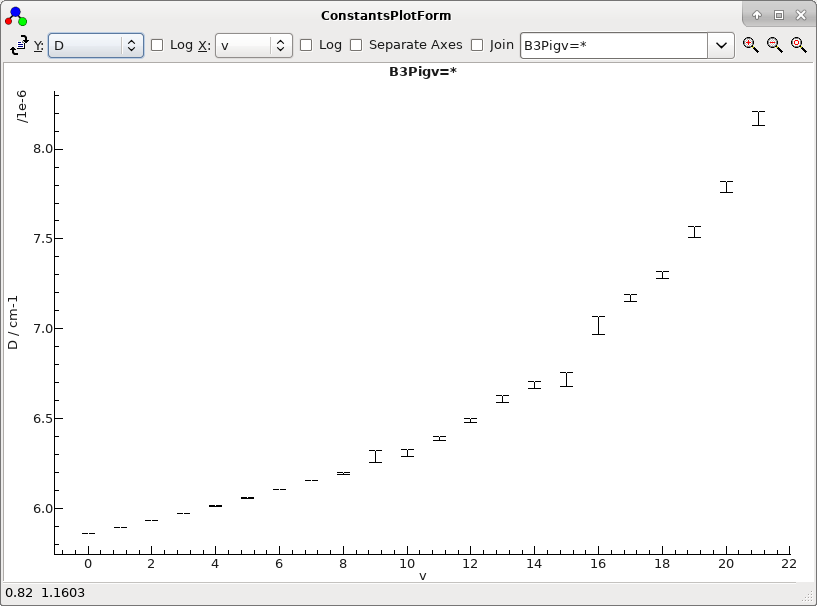Hovering the mouse over a point will show the underlying state and value. Right clicking on the plot will give a menu, including "Copy Table To Clipboard", which copies a table of the plotted values to the clipboard.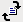Replot constants.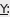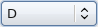Select constant to plot. This list is populated with all non-zero parameters from the selected states, and an "All" entry.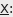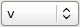Select the x axis; possible values are: Index: Running number for the plotted states, starting from 1 Sub-Index - index within manifold, starting from 1 v - vibrational quantum number, taking it from the last number in each state name. Origin - the state Origin. Offset - the offset of the origin from the lowest state in the manifold.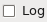Take Log10(Abs(value))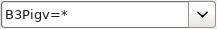Select the states to plot. A "*" is used to indicate the pattern of identified quantum numbers.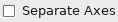If checked, use a separate axis for each type of constant; the vertical scale is shown as 0.0 - 1.0.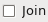Join constants for each state when plotting.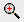Zoom to region selected by mouse.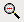Shrink plot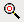Reset plot zoom to fill window